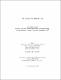## The signs in an elliptic net2014
Kumar, Manoj
##### Publisher
Lethbridge, Alta. : University of Lethbridge, Dept. of Mathematics and Computer Science
##### Abstract
Let R be an integral domain and let A be a finitely generated free abelian group. An elliptic net is a map form A to R, which satisfy a non linear recurrence relation. In this thesis we give a formula to compute the sign of any term of an elliptic net without actually computing the value of that term.
##### Keywords
Number Theory , Elliptic Nets , Mathematics